In spite of diligent planning, documentation, and proper process adherence in software development, occurrences of defects are inevitable. In today’s cutting edge competition, it is important to make conscious efforts to control and minimize these defects by using techniques to allow in-process quality monitoring and control. Defect Prediction using Rayleigh’s distribution curve is one such method that helps us to understand the density of the defects and their distribution across project phases as a project progresses.

Rayleigh’s defect prediction model is useful in determining the number of defects that are likely to occur during execution of a project in the early stages. This model can evolve and improve over time by considering data from previous phases of the project cycle. Predicting the total number of defects before testing begins improves the quality of the product being delivered and helps in planning and decision making for future project releases.

### Deriving Rayleigh’s Defect Prediction Curve

Rayleigh prediction requires two inputs: the number of phases planned and the total end-to-end defects. The model then predicts the number of defects in each phase. The Rayleigh function is given by the following formula: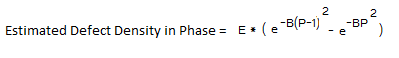Where E= Total no. of Defects

P= Phase Number

B= Defect Detection Efficiency

Defect Detection Efficiency can be calculated by using the following method: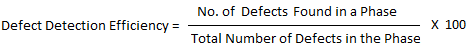Using the above formula the Rayleigh’s Curve can be plotted as given below: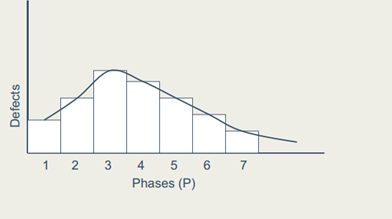Case 1: If the actual defects are less than the predicted defects, the quality of reviews need to be examined.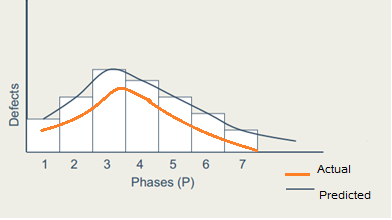Case 2: If the actual defects are more than the predicted, the model and total defects expected are to be readjusted dynamically.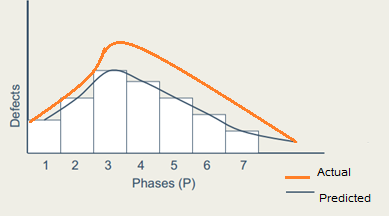### Advantages of Rayleigh’s Defect Prediction Model

• It is one of the dynamic methods to predict the reliability of the software.
• Only a few input parameters are required for the prediction process.
• These models are based on statistical distribution of defects found, which is proven to be better than the static models.
• Rayleigh’s Model is better than other dynamic models such as Exponential and S-Curve Models since it models the entire software development lifecycle.

Since the models and approaches vary depending on many factors, none of them will predict exact defect numbers. Rayleigh’s Model is the most commonly used and useful defect prediction model as it can be used to predict the additional test effort required to achieve desired quality level (as measured by number of remaining defects) during the testing phase. At the end of the testing phase, the model can be used to predict the number of remaining defects in the system and helping to predict reliability of the software.

Have you used Rayleigh’s Model? What did you think of it? What other models have you used? Reply to this post with your thoughts!